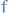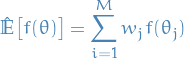# Approximate Bayesian Computation (ABC)

## Notation

•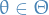parameters
•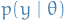generated samples from model with parameters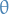•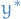denotes observed data
•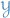is the domain of the observations
•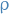is a metric on•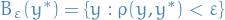## Overview

• Cases where computing the likelihood of the observed datais intractable
• ABC uses approximation of the likelihood obtained from simulation

## Rejection ABC

Let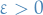be a similarity threshold, andbe the notion of distance, e.g. premetric on domainof observations.

The rejection ABC proceeds as follows:

1. Sample multiple model parameters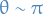.
2. For each, generate psuedo-dataset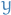from3. For each psuedo-datset, if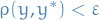, accept the generated, otherwise reject.

Result: Exact sample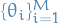from approximated posterior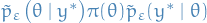, where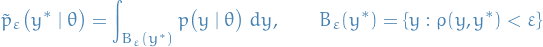Choice ofis crucial in the design of a n accurate ABC algorithm.

## Soft ABC

One can interpret the approximate likelihood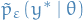in rejection ABC as the convolution of the true likelihoodand the "similarity" kernel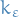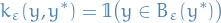In fact, one can use any similarity kernel parametrised by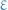satisfying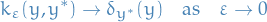which gives rise to the Soft ABC methods:

Soft ABC is an extension of rejection ABC which instead weights the parameter samples from the model instead of rejecting or accepting.

An example is using the Gaussian kernel: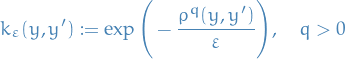Which results in the weighted sample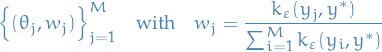which can be directly utilized in estimating posterior expectations, i.e. for a test function